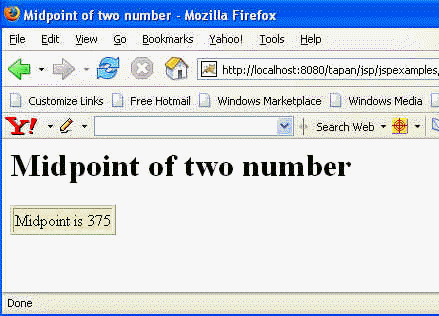Tutorials

# Midpoint of two number

While solving any mathematical problem it becomes important to find the number that is exactly between two numbers.

While solving any mathematical problem it becomes important to find the number that is exactly between two numbers.

# Midpoint of two number

While solving any mathematical problem it becomes important to find the number that is exactly between two numbers.

In this example we are finding a midpoint of two numbers by using the while loop.

The code of the program is given below:

 ``` Midpoint of two number ``` ``` ``` ```

Midpoint of two number

<% short a,b; a=250; b=500; while(++a < --b); out.println("Midpoint is "+a); %>
```

Output of the Program: